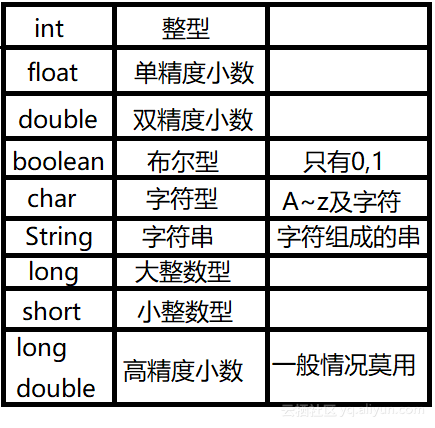Java零基础入门学习2：变量与变量类型

0
0
01. 云栖社区>
2. 博客>
3. 正文

Java零基础入门学习2：变量与变量类型1.初始化赋值

2.输入赋值

3.计算赋值int x;
x=1;

int x=1;

int a=1,b=2,c=3;

int a=1.1;

int a=1.0;

int a=2,b=5,c;
c=a+b;
System.out.println(c);

a=2,b=5,c=a+b,于是我们可以计算出c=7.

7+ 关注

corcosa 16052人浏览